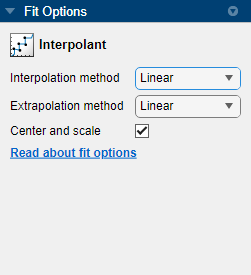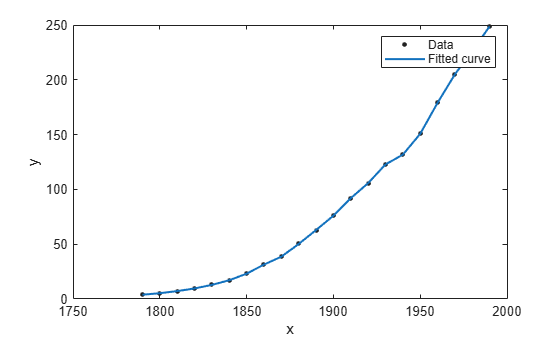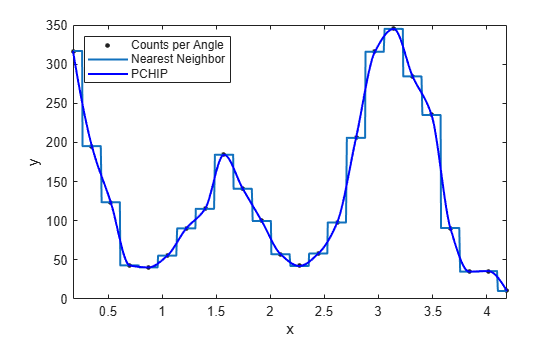## Interpolation with Curve Fitting Toolbox

Interpolation is a process for estimating values that lie between known data points.

Interpolation involves creating of a function that matches given data values yi at given data sites xi where f(xi) = yi, for all i.

Most interpolation methods create the interpolant f as the unique function of the formula

`$f\left(x\right)=\sum _{j}{f}_{j}\left(x\right){a}_{j},$`

where the form of the functions fj depends on the interpolation method.

For spline interpolation, the fj are the n consecutive B-splines Bj(x) = B(x|tj,...,tj+k), j = 1:n, of order k for a knot sequence t1 ≤ t2 ≤ ... ≤ tn + k.

Curve Fitting Toolbox™ supports the interpolation methods described in the following table.

Method

Description

Linear

Linear interpolation. This method fits a different linear polynomial between each pair of data points for curves, or between sets of three points for surfaces.

Nearest neighbor

Nearest neighbor interpolation. This method sets the value of an interpolated point to the value of the nearest data point.

Cubic spline

Cubic spline interpolation. This method fits a different cubic polynomial between each pair of data points for curves, or between sets of three points for surfaces.

Shape-preserving (PCHIP)

Piecewise cubic Hermite interpolation (PCHIP). This method preserves monotonicity and the shape of the data (for curves only).

Biharmonic (v4)

MATLAB® 4 `griddata` method. This method fits surfaces that also extrapolate well (for surfaces only).

Thin-plate spline

Thin-plate spline interpolation. This method fits smooth surfaces that also extrapolate well (for surfaces only).

Interpolant surface fits use the MATLAB function `scatteredInterpolant` for the linear and nearest neighbor methods, and the MATLAB function `griddata` for the cubic spline and biharmonic methods. The thin-plate spline method uses the `tpaps` function.

The interpolant method you use depends on several factors, including the characteristics of the data being fit, the required smoothness of the curve, speed considerations, and post-fit analysis requirements. The linear and nearest neighbor methods fit models efficiently, and the resulting curves are not very smooth. The cubic spline, shape-preserving, and biharmonic methods take longer to fit models, and the resulting curves are very smooth.

For example, the following plot shows a nearest neighbor interpolant fit and a shape-preserving (PCHIP) interpolant fit for the nuclear reaction data from the `carbon12alpha.mat` sample data set. The nearest neighbor interpolant is not as smooth as the shape-preserving interpolant.Note

Goodness-of-fit statistics, prediction bounds, and weights are not defined for interpolants. Additionally, the fit residuals are always 0 (within computer precision) because interpolants pass through the data points.

Biharmonic interpolant fits consist of radial basis function interpolants. All other interpolants supported by Curve Fitting Toolbox are piecewise polynomials and consist of multiple polynomials defined between data points. For cubic spline and PCHIP interpolation, four coefficients describe each piece. Curve Fitting Toolbox uses a cubic (third-degree) polynomial to calculate the four coefficients. Refer to the following for more information:

• `spline` for cubic spline interpolation

• `pchip` for shape-preserving (PCHIP) interpolation, and for a comparison of PCHIP and cubic spline interpolation

• `scatteredInterpolant`, `griddata`, and `tpaps` for surface interpolation

It is possible to fit a single polynomial interpolant to data, with a degree one less than the number of data points. However, the behavior of such fits is unpredictable between data points. Piecewise polynomials with lower-order segments do not diverge significantly from the fitting data domain, so they are useful for analyzing a wider range of data sets.

### Selecting an Interpolant Fit

#### Select Interpolant Fit Interactively

Open the Curve Fitter app by entering `curveFitter` at the MATLAB command line. Alternatively, on the Apps tab, in the Math, Statistics and Optimization group, click Curve Fitter.

On the Curve Fitter tab, in the Fit Type section, select an Interpolant fit. The app fits an interpolating curve or surface that passes through every data point.

In the Fit Options pane, you can specify the Interpolation method value.For curve data, you can set Interpolation method to `Linear`, `Nearest neighbor`, `Cubic Spline`, or ```Shape-preserving (PCHIP)```. For surface data, you can set Interpolation method to `Nearest neighbor`, `Linear`, `Cubic Spline`, `Biharmonic (v4)`, or ```Thin-plate spline```.

For surfaces, the Interpolant fit uses the `scatteredInterpolant` function for the `Linear` and `Nearest neighbor` methods, the `griddata` function for the `Cubic Spline` and `Biharmonic (v4)` methods, and the `tpaps` function for the `Thin-plate spline` method. Try the ```Thin-plate spline``` method when you require both smooth surface interpolation and good extrapolation properties.

Tip

If your data variables have very different scales, select and clear the Center and scale check box to see the difference in the fit. Normalizing the inputs can influence the results of the piecewise `Linear` and ```Cubic Spline``` interpolation, and ```Nearest neighbor``` surface interpolation methods.

#### Fit Linear Interpolant Model Using the `fit` Function

Load the `census` sample data set.

`load census`

The variables `pop` and `cdate` contain data for the population size and the year the census was taken, respectively.

You can use the `fit` function to fit any of the interpolant models described in Interpolant Model Names. In this case, fit a linear interpolant model using the `'linearinterp'` option, and then plot the result.

```f = fit(cdate,pop,'linearinterp'); plot(f,cdate,pop);```Compare Linear Interpolant Models

Load the `carbon12alpha` sample data set. Create both nearest neighbor and PCHIP interpolant fits using the `'nearestinterp'` and `'pchip'` options.

```load carbon12alpha f1 = fit(angle,counts,'nearestinterp'); f2 = fit(angle,counts,'pchip');```

Compare the fitted curves `f1` and `f2` by plotting them in the same figure.

```p1 = plot(f1,angle,counts); xlim([min(angle),max(angle)]) hold on p2 = plot(f2,'b'); hold off legend([p1;p2],'Counts per Angle','Nearest Neighbor','PCHIP',... 'Location','northwest')```